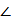# The transconductance of the MOSFET is:

1.  0.75 mS

2.  1 mS

3.  2 mS

4.  10 mS

4

1 mS

Explanation :
No Explanation available for this question

# The voltage gain of the amplifier is:

1.  +5

2.  -7.5

3.  +10

4.  -10

4

-10

Explanation :
No Explanation available for this question

# The following is true

1.  A finite signal is always bounded

2.  A bounded signal always possesses finite energy

3.  A bounded signal is always zero outside the interval [- t0, t0] for some t0

4.  A bounded signal is always finite

4

A bounded signal always possesses finite energy

Explanation :
No Explanation available for this question

# x (t) is a real valued function of a real variable with period T.Its trigonometric Fourier Series expansion contains no terms of frequency ω=2π (2k)/T;k =1, 2,.... Also,no sine terms are present.Then x (t) satisfies the equation

1.  x(t)=-x (t-T)

2.  x(t)=x(T-t)=-x(-t)

3.  x(t)=x(T-t)=-x (t-T/2)

4.  x(t)=x(t-T)=x(t-T/2)

4

x(t)=x(t-T)=x(t-T/2)

Explanation :
No Explanation available for this question

# In the figure the current source is 10 A,R=1 ,the impedances are ZC=- j?,and Z1=2j?. The Thevenin equivalent looking into the circuit across X-Y is

1.  √2 0V,(1 + 2j)

2.  2 45° V,(1-2j) ?

3.  2 45° V, (1 + j) ?

4.  √2 45° V, (1 + j) ?

4

√245° V, (1 + j) ?

Explanation :
No Explanation available for this question

# The three limbed non ideal core shown in the figure has three windings with nominal inductances Leach when measured individually with a single phase AC source.The inductance of the windings as connected will be

1.  very low

2.  L/3

3.  3L

4.  very high

4

very low

Explanation :
No Explanation available for this question

# Which of the following statements holds for the divergence of electric and magnetic flux densities

1.  Both are zero

2.  These are zero for static densities but non zero for time varying densities

3.  It is zero for the electric flux density

4.  It is zero for the magnetic flux density

4

It is zero for the magnetic flux density

Explanation :
No Explanation available for this question

# In transformers,which of the following statements is valid

1.  In an open circuit test,copper losses are obtained while in short circuit test,core losses are obtained

2.  In an open circuit test,current is drawn at high power factor

3.  In a short circuit test,current is drawn at zero power factor

4.  In an open circuit test,current is drawn at low power factor

4

Explanation :
No Explanation available for this question

# In transformers,which of the following statements is valid

1.  In an open circuit test,copper losses are obtained while in short circuit test core losses are obtained

2.  In an open circuit test,current is drawn at high power factor

3.  In a short circuit test,current is drawn at zero power factor

4.  In an open circuit test,current is drawn at low power factor

4

In an open circuit test,current is drawn at low power factor

Explanation :
No Explanation available for this question

# For a single phase capacitor start induction motor which of the following statements is valid

1.  The capacitor is used for power factor improvement

2.  The direction of rotation can be changed by reversing the main winding terminals

3.  The direction of rotation cannot be changed

4.  The direction of rotation can be changed by interchanging the supply terminals

4